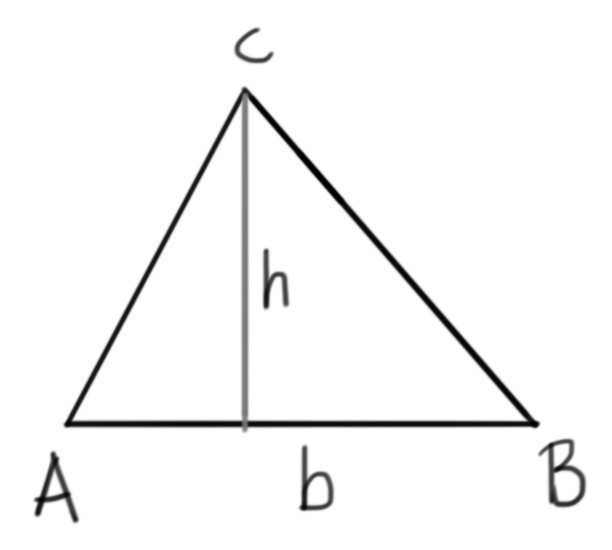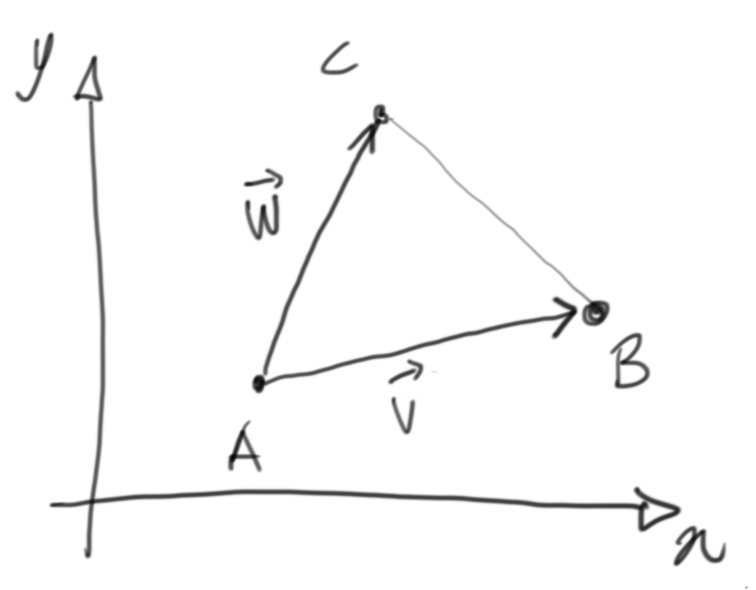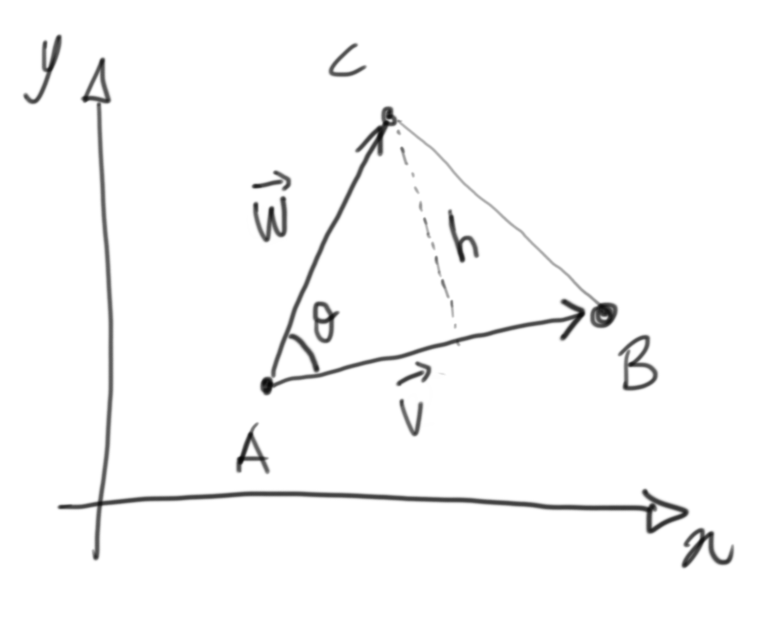TL;DR

I was thinking about calculating the area of a triangle…

… for reasons that go beyond this post, and I obviously started from the basic formula we were all teached in school: base times height divided by two. So with reference to the following picture:we have that segment $\overline{AB}$ is the base $b$ and the height with respect to it is h. In this case, then, we have that area $S$ is:

$S = \frac{b \cdot h}{2}$

Easy, right? Now let’s assume that you are given a triangle by its coordinates in the cartesian plane $XY$:In this case, we are given the three points:

$\mathbf{A} = (A_x, A_y) \\ \mathbf{B} = (B_x, B_y) \\ \mathbf{C} = (C_x, C_y)$

How to calculate the area in this case? Well…

# First of all, let’s switch to vectors

It’s easy to see that vectors $\vec{v}$ and $\vec{w}$ actually are an arrowized version of the two sides $\overline{AB}$ and $\overline{AC}$ respectively. This means that wherever we place these two vectors applied to a point in the plane, it will be the same triangle moved around and the area will be the same.

Long story short… we will work with these two vectors:

$\vec{v} = (v_x, v_y) = (B_x - A_x, B_y - A_y) \\ \vec{w} = (w_x, w_y) = (C_x - A_x, C_y - A_y)$

# How to calculate the area, then?

Let’s just get back to the origins…The base $b$ is just the length of vector $\vec{v}$, which we will represent like this:

$b = |v| = \sqrt{v_x^2 + v_y^2}$

Now the corresponding height $h$ can be calculated like this:

$h = |w| \cdot sin\theta = \sqrt{w_x^2 + w_y^2} \cdot sin\theta$

So… the area $S$ will be:

$S = \frac{b \cdot h}{2} = \frac{|v|\cdot |w| \cdot sin\theta}{2}$

# So what’s with this angle?!?

It turns out that you can calculate the scalar product of the two vectors in a pretty straightforward way, and it is indeed related to the cosine of the angle $\theta$:

$\vec{v}\cdot\vec{w} = v_x \cdot w_x + v_y \cdot w_y = |v| \cdot |w| \cdot cos\theta$

which means:

$cos\theta = \frac{\vec{v}\cdot\vec{w}}{|v| \cdot |w|}$

Now we just have to remember Pythagora’s theorem:

$sin^2\theta + cos^2\theta = 1 \\ sin^2\theta = 1 - cos^2\theta \\ sin^2\theta = 1 - \frac{(\vec{v}\cdot\vec{w})^2}{|v|^2 \cdot |w|^2} \\ sin^2\theta = \frac{|v|^2 \cdot |w|^2 - (\vec{v}\cdot\vec{w})^2}{|v|^2 \cdot |w|^2}$

We will concentrate on the positive value for the sine (so that we get positive areas), so:

$sin\theta = \frac{\sqrt{|v|^2 \cdot |w|^2 - (\vec{v}\cdot\vec{w})^2}}{|v| \cdot |w|}$

Let’s multiply by the denominator on both sides… and divide by two as well:

$\frac{|v| \cdot |w| \cdot sin\theta}{2} = \frac{\sqrt{|v|^2 \cdot |w|^2 - (\vec{v}\cdot\vec{w})^2}}{2}$

Now wait! The left hand side is our area $S$, so…

$S = \frac{\sqrt{|v|^2 \cdot |w|^2 - (\vec{v}\cdot\vec{w})^2}}{2} \\ S = \frac{\sqrt{(v_x^2 + v_y^2) \cdot (w_x^2 + w_y^2) - (v_x \cdot w_x + v_y \cdot w_y)^2}}{2}$

# Did you notice?

The scalar(dot) product of a generic vector $\vec{v}$ with itself ends up being the square of its length:

$\vec{v}\cdot\vec{v} = |v|^2$

so our area can also be expressed as:

$S = \frac{ \sqrt{ (\vec{v}\cdot\vec{v})\cdot(\vec{w}\cdot\vec{w}) - (\vec{v}\cdot\vec{w})\cdot(\vec{v}\cdot\vec{w}) } }{2}$

Nifty, uh?!?

# It’s easier with some code

After this long ride, let’s just take a look at some code. We assume that our points are represented by arrays (references) with $X$ coordinate at index 0 and $Y$ coordinate at index 1;

sub triangle_area {
my ($A,$B, $C) = @_; my ($v_x, $v_y) = map {$B->[$_] -$A->[$_]} 0 .. 1; my ($w_x, $w_y) = map {$C->[$_] -$A->[$_]} 0 .. 1; my$vv = $v_x *$v_x + $v_y *$v_y;
my $ww =$w_x * $w_x +$w_y * $w_y; my$vw = $v_x *$w_x + $v_y *$w_y;
return sqrt($vv *$ww - $vw *$vw) / 2;
}


And I guess we’re done for today!

Comments? Octodon, , GitHub, Reddit, or drop me a line!